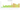# тңг.

## Kazakhstani Tenge

Kazakhstan's official currency is the tenge. There are 100 tiyn in it.

#### how has the value of the currency changed in the last year?## USD 1 = KZT 461.65

How much is10 US dollars worth inKazakhstani tenges?
At the current exchange rate, 10 US dollars is worth 4,616.55 Kazakhstani tenges
How much is50 US dollars worth inKazakhstani tenges?
At the current exchange rate, 50 US dollars is worth 23,082.73 Kazakhstani tenges
How much is100 US dollars worth inKazakhstani tenges?
At the current exchange rate, 100 US dollars is worth 46,165.46 Kazakhstani tenges
How much is500 US dollars worth inKazakhstani tenges?
At the current exchange rate, 500 US dollars is worth 230,827.32 Kazakhstani tenges
How much is2,000 US dollars worth inKazakhstani tenges?
At the current exchange rate, 2,000 US dollars is worth 923,309.28 Kazakhstani tenges
How much is10 US dollars worth inKazakhstani tenges?
At the current exchange rate, 10 US dollars is worth 4,616.55 Kazakhstani tenges
How much is50 US dollars worth inKazakhstani tenges?
At the current exchange rate, 50 US dollars is worth 23,082.73 Kazakhstani tenges
How much is100 US dollars worth inKazakhstani tenges?
At the current exchange rate, 100 US dollars is worth 46,165.46 Kazakhstani tenges
How much is500 US dollars worth inKazakhstani tenges?
At the current exchange rate, 500 US dollars is worth 230,827.32 Kazakhstani tenges
How much is2,000 US dollars worth inKazakhstani tenges?
At the current exchange rate, 2,000 US dollars is worth 923,309.28 Kazakhstani tenges